💬 👋 We’re always here. Join our Discord to connect with other students 24/7, any time, night or day.Join Here!

## Educators

RkssRG
+ 17 more educators

### Problem 1

Find the volume of the solid obtained by rotating the region bounded by the given curves about the specified line. Sketch the region, the solid, and a typical disk or washer.

$y = x + 1$ , $y = 0$ , $x = 0$ , $x = 2$ ; about the x-axisCarson M.

### Problem 2

Find the volume of the solid obtained by rotating the region bounded by the given curves about the specified line. Sketch the region, the solid, and a typical disk or washer.

$y = \frac{1}{x}$ , $y = 0$ , $x = 1$ , $x = 4$ ; about the x-axis### Problem 3

Find the volume of the solid obtained by rotating the region bounded by the given curves about the specified line. Sketch the region, the solid, and a typical disk or washer.

$y = \sqrt{x - 1}$ , $y = 0$ , $x = 5$ ; about the x-axisChris T.

### Problem 4

Find the volume of the solid obtained by rotating the region bounded by the given curves about the specified line. Sketch the region, the solid, and a typical disk or washer.

$y = e^x$ , $y = 0$ , $x = -1$ , $x = 1$ ; about the x-axisChris T.

### Problem 5

Find the volume of the solid obtained by rotating the region bounded by the given curves about the specified line. Sketch the region, the solid, and a typical disk or washer.

$x = 2 \sqrt{y}$ , $x = 0$ , $y = 9$ ; about the y-axis

RG
Raymond G.

### Problem 6

Find the volume of the solid obtained by rotating the region bounded by the given curves about the specified line. Sketch the region, the solid, and a typical disk or washer.

$2x = y^2$ , $x = 0$ , $y = 4$ ; about the y-axisChris T.

### Problem 7

Find the volume of the solid obtained by rotating the region bounded by the given curves about the specified line. Sketch the region, the solid, and a typical disk or washer.

$y = x^3$ , $y = x$ , $x \ge 0$ ; about the x-axis

RG
Raymond G.

### Problem 8

Find the volume of the solid obtained by rotating the region bounded by the given curves about the specified line. Sketch the region, the solid, and a typical disk or washer.

$y = 6 - x^2$ , $y = 2$ ; about the x-axisChris T.

### Problem 9

Find the volume of the solid obtained by rotating the region bounded by the given curves about the specified line. Sketch the region, the solid, and a typical disk or washer.

$y^2 = x$ , $x = 2y$ ; about the y-axis

RG
Raymond G.

### Problem 10

Find the volume of the solid obtained by rotating the region bounded by the given curves about the specified line. Sketch the region, the solid, and a typical disk or washer.

$x = -2 - y^2$ , $x = y^4$ ; about the y-axisChris T.

### Problem 11

Find the volume of the solid obtained by rotating the region bounded by the given curves about the specified line. Sketch the region, the solid, and a typical disk or washer.

$y = x^2$ , $x = y^2$ ; about $y = 1$Chris T.

### Problem 12

Find the volume of the solid obtained by rotating the region bounded by the given curves about the specified line. Sketch the region, the solid, and a typical disk or washer.

$y = x^3$ , $y = 1$ , $x = 2$ ; about $y = -3$Chris T.

### Problem 13

Find the volume of the solid obtained by rotating the region bounded by the given curves about the specified line. Sketch the region, the solid, and a typical disk or washer.

$y = 1 + \sec x$ , $y = 3$ ; about $y = 1$Chris T.

### Problem 14

Find the volume of the solid obtained by rotating the region bounded by the given curves about the specified line. Sketch the region, the solid, and a typical disk or washer.

$y = \sin x$ , $y = \cos x$ , $0 \le x \le \frac{\pi}{4}$ ; about $y = -1$Chris T.

### Problem 15

Find the volume of the solid obtained by rotating the region bounded by the given curves about the specified line. Sketch the region, the solid, and a typical disk or washer.

$y = x^3$ , $y = 0$ , $x = 1$ ; about $x = 2$Chris T.

### Problem 16

Find the volume of the solid obtained by rotating the region bounded by the given curves about the specified line. Sketch the region, the solid, and a typical disk or washer.

$xy = 1$ , $y = 0$ , $x = 1$ , $x = 2$ ; about $x = -1$Chris T.

### Problem 17

Find the volume of the solid obtained by rotating the region bounded by the given curves about the specified line. Sketch the region, the solid, and a typical disk or washer.

$x = y^2$ , $x = 1 - y^2$ ; about $x = 3$Chris T.

### Problem 18

Find the volume of the solid obtained by rotating the region bounded by the given curves about the specified line. Sketch the region, the solid, and a typical disk or washer.
$$y=x, y=0, x=2, x=4 ; \quad \text { about } x=1$$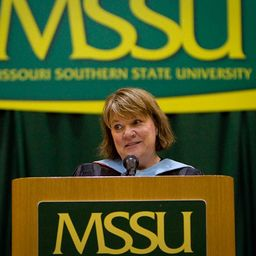Linda H.

### Problem 19

Refer to the figure and find the volume generated by rotating the given region about the specified line.

$\Re_1$ about $OA$Mutahar M.

### Problem 20

Refer to the figure and find the volume generated by rotating the given region about the specified line.

$\Re_1$ about $OC$Chris T.

### Problem 21

Refer to the figure and find the volume generated by rotating the given region about the specified line.

$\Re_1$ about $AB$Chris T.

### Problem 22

Refer to the figure and find the volume generated by rotating the given region about the specified line.

$\Re_1$ about $BC$Jacquelyn T.

### Problem 23

Refer to the figure and find the volume generated by rotating the given region about the specified line.

$\Re_2$ about $OA$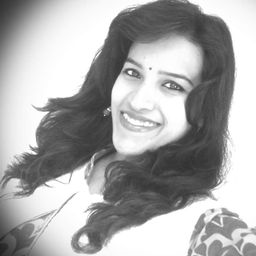Aparna S.

### Problem 24

Refer to the figure and find the volume generated by rotating the given region about the specified line.

$\Re_2$ about $OC$Chris T.

### Problem 25

Refer to the figure and find the volume generated by rotating the given region about the specified line.

$\Re_2$ about $AB$Chris T.

### Problem 26

Refer to the figure and find the volume generated by rotating the given region about the specified line.

$\Re_2$ about $BC$Chris T.

### Problem 27

Refer to the figure and find the volume generated by rotating the given region about the specified line.

$\Re_3$ about $OA$Chris T.

### Problem 28

Refer to the figure and find the volume generated by rotating the given region about the specified line.

$\Re_3$ about $OC$Chris T.

### Problem 29

Refer to the figure and find the volume generated by rotating the given region about the specified line.

$\Re_3$ about $AB$Chris T.

### Problem 30

Refer to the figure and find the volume generated by rotating the given region about the specified line.

$\Re_3$ about $BC$Chris T.

### Problem 31

Set up an integral for the volume of the solid obtained by rotating the region bounded by the given curves about the specified line. Then use your calculator to evaluate the integral correct to five decimal places.

$y = e^{-x^2}$ , $y = 0$ , $x = -1$ , $x = 1$

(b) About $y = -1$

SO
Sari O.

### Problem 32

Set up an integral for the volume of the solid obtained by rotating the region bounded by the given curves about the specified line. Then use your calculator to evaluate the integral correct to five decimal places.

$y = 0$ , $y = \cos^2 x$ , $\frac{-\pi}{2} \le x \le \frac{\pi}{2}$

(b) About $y = 1$Carson M.

### Problem 33

Set up an integral for the volume of the solid obtained by rotating the region bounded by the given curves about the specified line. Then use your calculator to evaluate the integral correct to five decimal places.

$x^2 + 4y^2 = 4$

(b) About $y = 2$
(b) About $x = 2$Linda H.

### Problem 34

Set up an integral for the volume of the solid obtained by rotating the region bounded by the given curves about the specified line. Then use your calculator to evaluate the integral correct to five decimal places.

$y =x^2$ , $x^2 + y^2 = 1$ , $y \ge 0$Chris T.

### Problem 35

Use a graph to find approximate x-coordinates of the points of intersection of the given curves. Then use your calculator to find (approximately) the volume of the solid obtained by rotating about the x-axis the region bounded by these curves.

$y = \ln (x^6 + 2)$ , $y = \sqrt{3 - x^3}$Chris T.

### Problem 36

Use a graph to find approximate x-coordinates of the points of intersection of the given curves. Then use your calculator to find (approximately) the volume of the solid obtained by rotating about the x-axis the region bounded by these curves.

$y = 1 + xe^{-x^3}$ , $y = \arctan x^2$Chris T.

### Problem 37

Use a computer algebra system to find the exact volume of the solid obtained by rotating the region bounded by the given curves about the specified line.

$y = \sin^2 x$ , $y = 0$ , $0 \le x \le \pi$ ; about $y = -1$Aparna S.

### Problem 38

Use a computer algebra system to find the exact volume of the solid obtained by rotating the region bounded by the given curves about the specified line.

$y = x$ , $y = xe^{1 - \frac{x}{2}}$ ; about $y = 3$Chris T.

### Problem 39

Each integral represents the volume of a solid. Describe the solid.

$\pi \displaystyle \int_{0}^\pi \sin x dx$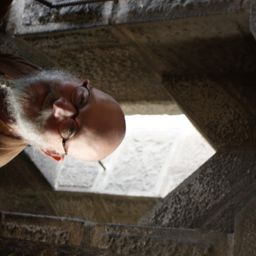Michael C.

### Problem 40

Each integral represents the volume of a solid. Describe the solid.

$\pi \displaystyle \int_{-1}^1 (1 -y^2)^2 dy$Yuki H.

### Problem 41

Each integral represents the volume of a solid. Describe the solid.

$\pi \displaystyle \int_{0}^1 (y^4 - y^8) dy$Linda H.

### Problem 42

Each integral represents the volume of a solid. Describe the solid.

$\pi \displaystyle \int_{1}^4 [3^2 - (3 - \sqrt{x})^2] dx$Amrita B.

### Problem 43

A CAT scan produces equally spaced cross-sectional views of a human organ that provide information about the organ otherwise obtained only by surgery. Suppose that a CAT scan of a human liver shows cross-sections spaced 1.5 cm apart. The liver is 15 cm long and the cross-sectional areas, in square centimeters, are 0, 18, 58, 79, 94, 106, 117, 128, 63, 39, and 0. Use the Midpoint Rule to estimate the volume of the liver.

AL
Andrew L.

### Problem 44

A log 10 m long is cut at 1-meter intervals and its cross- sectional areas $A$ (at a distance x from the end of the log) are listed in the table. Use the Midpoint Rule with $n = 5$ to estimate the volume of the log.Amrita B.

### Problem 45

(a) If the region shown in the figure is rotated about the x-axis to form a solid, use the Midpoint Rule with $n = 4$ to estimate the volume of the solid.
(b) Estimate the volume if the region is rotated about the y-axis. Again use the Midpoint Rule with $n = 4$.Linda H.

### Problem 46

(a) A model for the shape of the bird's egg is obtained by rotating about the x-axis the region under the graph of
$$f(x) = (ax^3 + bx^2 + cx + d) \sqrt{1 - x^2}$$
Use $CAS$ to find the volume of such an egg.
(b) For a red-throated loon, $a = -0.06$, $b = 0.04$, $c = 0.1$, and $d = 0.54$. Graph $f$ and find the volume of an egg of this species.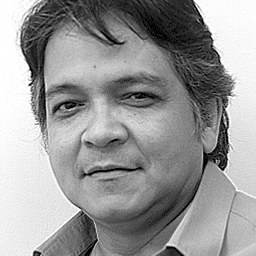Oswaldo J.

### Problem 47

Find the volume of the described solid $S$.
A right circular cone with height $h$ and base radius $r$.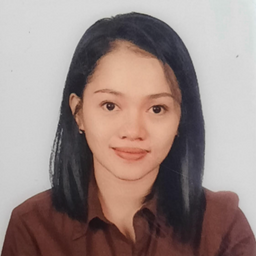Ma. Theresa A.

### Problem 48

Find the volume of the described solid $S$.
A frustum of a right circular cone with height $h$, lower base radius $R$, and top radius $r$.Chris T.

### Problem 49

Find the volume of the described solid $S$.
A cap of a sphere with radius $r$ and height $h$.Chris T.

### Problem 50

Find the volume of the described solid $S$.
A frustum of a pyramid with square base of side $b$, square top of side $a$, and height $h$

What happens if $a = b$? What happens if $a = 0$?Cindy R.

### Problem 51

Find the volume of the described solid $S$.
A pyramid with height $h$ and rectangular base with dimensions $b$ and $2b$.Amrita B.

### Problem 52

Find the volume of the described solid $S$.
A pyramid with height $h$ and base an equilateral triangle with side $a$ (a tetrahedron).Aparna S.

### Problem 53

Find the volume of the described solid $S$.
A tetrahedron with three mutually perpendicular faces and three mutually perpendicular edges with lengths 3 cm, 4 cm, and 5 cm.Chris T.

### Problem 54

Find the volume of the described solid $S$.
The base of $S$ is a circular disk with radius $r$. Parallel cross sections perpendicular to the base are squares.Chris T.

### Problem 55

Find the volume of the described solid $S$.
The base of $S$ is an elliptical region with boundary curve $9x^2 + 4y^2 = 36$. Cross-sections perpendicular to the x-axis are isosceles right triangles with hypotenuse in the base.Chris T.

### Problem 56

Find the volume of the described solid $S$.
The base of $S$ is the triangular region with vertices $(0, 0)$, $(1, 0)$, and $(0, 1)$. Cross-sections perpendicular to the y-axis are equilateral triangles.Chris T.

### Problem 57

Find the volume of the described solid $S$.
The base of $S$ is the same base as in Exercise 56, but cross-sections perpendicular to the x-axis are squares.Jacquelyn T.

### Problem 58

Find the volume of the described solid $S$.
The base of $S$ is the region enclosed by the parabola $y = 1 - x^2$ and the x-axis. Cross-sections perpendicular to the y-axis are squares.Chris T.

### Problem 59

Find the volume of the described solid $S$.
The base of $S$ is the same base as in Exercise 58, but cross-sections perpendicular to the x-axis are isosceles triangles with height equal to the base.Chris T.

### Problem 60

Find the volume of the described solid $S$.
The base of $S$ is the region enclosed by $y = 2 - x^2$ and the x-axis. Cross-sections perpendicular to the y-axis are quarter-circles.Chris T.

### Problem 61

Find the volume of the described solid $S$.
The solid $S$ is bounded by circles that are perpendicular to the x-axis, intersect the x-axis, and have centers on the parabola $y = \frac{1}{2} (1 - x^2)$, $-1 \le x \le 1$.Michael C.

### Problem 62

The base of $S$ is a circular disk with radius $r$. Parallel cross-sections perpendicular to the base are isosceles triangles with height $h$ and unequal side in the base.
(a) Set up an integral for the volume of $S$.
(b) By interpreting the integral as an area, find the volume of $S$.Carson M.

### Problem 63

(a) Set up an integral for the volume of a solid torus (the donut-shaped solid shown in the figure) with radii $r$ and $R$.
(b) By interpreting the integral as an area, find the volume of the torus.Carson M.

### Problem 64

Solve Example 9 taking cross-sections to be parallel to the line of intersection of the two planes.Chris T.

### Problem 65

(a) Cavalieri's Principle states that if a family of parallel planes gives equal cross-section areas for two solids $S_1$ and $S_2$ then the volumes of $S_1$ and $S_2$ are equal. Prove this principle.
(b) Use Cavalieri's Principle to find the volume of the oblique cylinder shown in the figure.Carson M.

### Problem 66

Find the volume common to two circular cylinders, each with radius $r$, if the axes of the cylinders intersect at right angles.Amrita B.

### Problem 67

Find the volume common to two spheres, each with radius $r$, if the center of each sphere lies on the surface of the other sphere.Eduard S.

### Problem 68

A bowl is shaped like a hemisphere with diameter 30 cm. A heavy ball with diameter 10 cm is placed in the bowl and water is poured into the bowl to a depth of $h$ centimeters. Find the volume of water in the bowl.

DM
David M.

### Problem 69

A hole of radius $r$ is bored through the middle of a cylinder of radius $R > r$ at right angles to the axis of the cylinder. Set up, but do not evaluate, an integral for the volume cut out.Aparna S.

### Problem 70

A hole of radius $r$ is bored through the center of a sphere of radius $R > r$. Find the volume of the remaining portion of the sphere.Carson M.

### Problem 71

Some of the pioneers of calculus, such as Kepler and Newton, were inspired by the problem of finding the
volumes of wine barrels. (In fact Kepler published a book Stereometria doliorum in 1615 devoted to methods for finding the volumes of barrels.) They often approximated the shape of the sides by parabolas.
(a) A barrel with height $h$ and maximum radius $R$ is constructed by rotating about the x-axis the parabola $y = R - cx^2$, $\frac{-h}{2} \le x \le \frac{h}{2}$, where c is a positive constant. Show that the radius of each end of the barrel is $r = R - d$, where $d = \frac{ch^2}{4}$.
(b) Show that the volume enclosed by the barrel is
$$V = \frac{1}{3} \pi h (2R^2 + r^2 - \frac{2}{5} d^2)$$Linda H.
Suppose that a region $\Re$ has area $A$ and lies above the x-axis. When $\Re$ is rotated about the x-axis, it sweeps out a solid with volume $V_1$. When $\Re$ is rotated about the line $y = -k$ (where $k$ is a positive number), it sweeps out a solid with volume $V_2$. Express $V_2$ in terms of $V_1$, $k$, and $A$.